# Convert fractions, percentages and decimals

1
Fraction to decimal
Choose correct, Multiple choice Difficult
2
Fraction to percent
Choose correct, Multiple choice
3
Decimal to fraction
Choose correct, Multiple choice
4
Decimal to percent
Choose correct, Multiple choice
5
Percent to fraction
Choose correct, Multiple choice
6
Percent to decimal
Choose correct, Multiple choice
7
Fraction to decimal
Write yourself, Questions with text answers
8
Fraction to percent
Write yourself, Questions with text answers
9
Decimal to fraction
Write yourself, Questions with text answers
10
Decimal to percent
Write yourself, Questions with text answers
11
Percent to fraction
Write yourself, Questions with text answers
12
Percent to decimal
Write yourself, Questions with text answers
Do you want to play locked game level?

## Recent activities in this game

Someone had a problem at level 1. Fraction to decimal (Choose correct) .
2023-12-08 19:59 after 12 seconds of playing.
Someone had a problem at level 1. Fraction to decimal (Choose correct) .
2023-12-06 05:03 after 1 second of playing.
Someone had a problem at level 1. Fraction to decimal (Choose correct) .
2023-12-06 04:55 after 1 second of playing.
Someone had a problem at level 3. Decimal to fraction (Choose correct) .
2023-12-06 04:14 after 0 seconds of playing.
Someone had a problem at level 4. Decimal to percent (Choose correct) .
2023-12-06 04:13 after 1 second of playing.
Someone had a problem at level 2. Fraction to percent (Choose correct) .
2023-12-06 04:01 after 0 seconds of playing.
Someone had a problem at level 8. Fraction to percent (Write yourself) .
2023-12-05 21:33 after 1 second of playing.
Someone had a problem at level 8. Fraction to percent (Write yourself) .
2023-12-05 21:33 after 0 seconds of playing.
Someone had a problem at level 8. Fraction to percent (Write yourself) .
2023-12-05 21:33 after 1 second of playing.
Someone had a problem at level 8. Fraction to percent (Write yourself) .
2023-12-05 21:33 after 0 seconds of playing.

Practice convert a number between fraction, percentage and decimal. Includes the ten steps from a whole of one-tenth.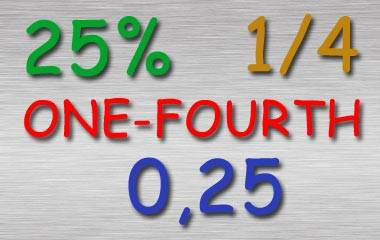## Instructions for Convert fractions, percentages and decimals

A number can be written in many ways. In this game you can exercise on writing it in fractional, decimal and percentage terms. You train full, half, third, fourth, fifth, sixth, seventh, eighth, ninth and tenth. Depending on the level above, you will practice on one of the following conversions: Fraction to percent, eg, 1/10 = 10% Fraction to decimal, eg 1/2 = 0.5 Percent to fraction 25% = 1/4 Percent to decimal, 33%? 0.33 Decimal to fraction, eg, 0.125 = 1/8 Decimal to percent, eg, 0.25 = 25% The fractions are rounded off in this game to decimals and percentages with two significant figures. The fractions will be varied between text and digits!

### 1. Fraction to decimal (Choose correct)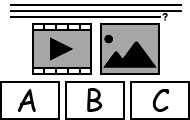Multiple choice - The level consists of 20 questions, but a maximum of 10 questions are practiced per game round. For each question, the player is given three possible answers. Only one of these is correct. It is up to the player to choose the correct alternative. The student has 3 hearts (extra lives) that keep the game round going. There is no time limit. When the student has selected all the correct answers, the game round is completed. If it is too difficult, the player can click the button named Cancel - then the correct answers will be displayed - and then try to complete the level again.

### 2. Fraction to percent (Choose correct)Multiple choice - The level consists of 20 questions, but a maximum of 10 questions are practiced per game round. For each question, the player is given three possible answers. Only one of these is correct. It is up to the player to choose the correct alternative. The student has 3 hearts (extra lives) that keep the game round going. There is no time limit. When the student has selected all the correct answers, the game round is completed. If it is too difficult, the player can click the button named Cancel - then the correct answers will be displayed - and then try to complete the level again.

### 3. Decimal to fraction (Choose correct)Multiple choice - The level consists of 20 questions, but a maximum of 10 questions are practiced per game round. For each question, the player is given two or three possible answers. Only one of these is correct. It is up to the player to choose the correct alternative. The student has 3 hearts (extra lives) that keep the game round going. There is no time limit. When the student has selected all the correct answers, the game round is completed. If it is too difficult, the player can click the button named Cancel - then the correct answers will be displayed - and then try to complete the level again.

### 4. Decimal to percent (Choose correct)Multiple choice - The level consists of 10 questions and all are practiced every game round. For each question, the player is given two or three possible answers. Only one of these is correct. It is up to the player to choose the correct alternative. The student has 3 hearts (extra lives) that keep the game round going. There is no time limit. When the student has selected all the correct answers, the game round is completed. If it is too difficult, the player can click the button named Cancel - then the correct answers will be displayed - and then try to complete the level again.

### 5. Percent to fraction (Choose correct)Multiple choice - The level consists of 20 questions, but a maximum of 10 questions are practiced per game round. For each question, the player is given three possible answers. Only one of these is correct. It is up to the player to choose the correct alternative. The student has 3 hearts (extra lives) that keep the game round going. There is no time limit. When the student has selected all the correct answers, the game round is completed. If it is too difficult, the player can click the button named Cancel - then the correct answers will be displayed - and then try to complete the level again.

### 6. Percent to decimal (Choose correct)Multiple choice - The level consists of 10 questions and all are practiced every game round. For each question, the player is given three possible answers. Only one of these is correct. It is up to the player to choose the correct alternative. The student has 3 hearts (extra lives) that keep the game round going. There is no time limit. When the student has selected all the correct answers, the game round is completed. If it is too difficult, the player can click the button named Cancel - then the correct answers will be displayed - and then try to complete the level again.

### 7. Fraction to decimal (Write yourself)Questions with text answers - The level consists of 20 questions, but a maximum of 10 questions are practiced per game round. The student has to write the correct answer without color guidance and has 3 attempts to write the correct word or sentence in each question's text field that are not case-sensitive. The student receives feedback on each keystroke to make it easier to type the correct answer. If the entered character is correct, the text turns green and if it's incorrect, the text turns red. There is no time limit. When the student has written all the answers correctly, the game round is completed. If it is too difficult, the player can click the button named Cancel - then the correct answers will be displayed - and then try to complete the level again.

### 8. Fraction to percent (Write yourself)Questions with text answers - The level consists of 20 questions, but a maximum of 10 questions are practiced per game round. The student has to write the correct answer without color guidance and has 3 attempts to write the correct word or sentence in each question's text field that are not case-sensitive. The student receives feedback on each keystroke to make it easier to type the correct answer. If the entered character is correct, the text turns green and if it's incorrect, the text turns red. There is no time limit. When the student has written all the answers correctly, the game round is completed. If it is too difficult, the player can click the button named Cancel - then the correct answers will be displayed - and then try to complete the level again.

### 9. Decimal to fraction (Write yourself)Questions with text answers - The level consists of 20 questions, but a maximum of 10 questions are practiced per game round. The student has to write the correct answer without color guidance and has 3 attempts to write the correct word or sentence in each question's text field that are not case-sensitive. The student receives feedback on each keystroke to make it easier to type the correct answer. If the entered character is correct, the text turns green and if it's incorrect, the text turns red. There is no time limit. When the student has written all the answers correctly, the game round is completed. If it is too difficult, the player can click the button named Cancel - then the correct answers will be displayed - and then try to complete the level again.

### 10. Decimal to percent (Write yourself)Questions with text answers - The level consists of 10 questions and all are practiced every game round. The student has to write the correct answer without color guidance and has 3 attempts to write the correct word or sentence in each question's text field that are not case-sensitive. The student receives feedback on each keystroke to make it easier to type the correct answer. If the entered character is correct, the text turns green and if it's incorrect, the text turns red. There is no time limit. When the student has written all the answers correctly, the game round is completed. If it is too difficult, the player can click the button named Cancel - then the correct answers will be displayed - and then try to complete the level again.

### 11. Percent to fraction (Write yourself)Questions with text answers - The level consists of 20 questions, but a maximum of 10 questions are practiced per game round. The student has to write the correct answer without color guidance and has 3 attempts to write the correct word or sentence in each question's text field that are not case-sensitive. The student receives feedback on each keystroke to make it easier to type the correct answer. If the entered character is correct, the text turns green and if it's incorrect, the text turns red. There is no time limit. When the student has written all the answers correctly, the game round is completed. If it is too difficult, the player can click the button named Cancel - then the correct answers will be displayed - and then try to complete the level again.

### 12. Percent to decimal (Write yourself)Questions with text answers - The level consists of 10 questions and all are practiced every game round. The student has to write the correct answer without color guidance and has 3 attempts to write the correct word or sentence in each question's text field that are not case-sensitive. The student receives feedback on each keystroke to make it easier to type the correct answer. If the entered character is correct, the text turns green and if it's incorrect, the text turns red. There is no time limit. When the student has written all the answers correctly, the game round is completed. If it is too difficult, the player can click the button named Cancel - then the correct answers will be displayed - and then try to complete the level again.

### Calculation of knowledge points

Each completed game level gives 1 knowledge point in Convert fractions, percentages and decimals. The maximum number of points (12 knowledge points) is achieved when you pass all 12 levels. You'll get a bronze medal when you complete a level 2 times and a silver medal after 5 completed rounds. A gold medal will be received after 10 completed rounds. In Convert fractions, percentages and decimals, the maximum number of collectable gold medals is 12. You only earn knowledge points for levels that has not been cleared before. A cleared level has a green background above. Even when you have completed a level, you can continue practicing at that level, but it gives you no more knowledge points.

Subject
Math
Target group
Practices
Fractions Percents Decimals Conversion
Game creator
Updated
2014-11-22
Published online
2014-11-22
Unique id
239
Saved by
0-5 users
Operating system
Browser
Price
Free of charge

3.9
Total 226
5
140
4
23
3
7
2
12
1
44

### Report

If you find a spelling mistake or something else that should be corrected, feel free to contact the game creator or us at Helpful Games.

Write a comment below to contact the game creator or

There are a total of 6 comments on this knowledge game.

N
8 November 2022 19:56
Great but could make the questions a bit more harder for the grade level they say they are.
18 February 2022 21:33
I mean the games are kinda hard but not a lot :)
A
8 October 2019 20:19
Hi my name is alhanoof and iam in grad 6 I would play this game in my free time becose its nice .
C
27 August 2019 00:32
this game helps take the stress off of a test I have tomorrow. I was freaking out not knowing what to do and helpful games came to the rescue. It cant get better than that!
W
7 March 2018 18:07
This game is awesome! You guys rock!!!!
F
7 March 2018 17:55
We love this game, and want to practice more decimals, and fractions!!!!!! Chimichanga!!!!!!!
Most played
1.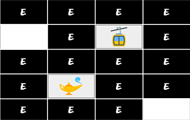Brain training
2.Brain training
3.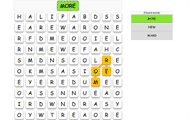English
4.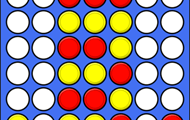Brain training
5.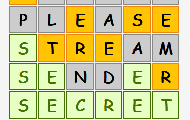English

More plus membership benefits